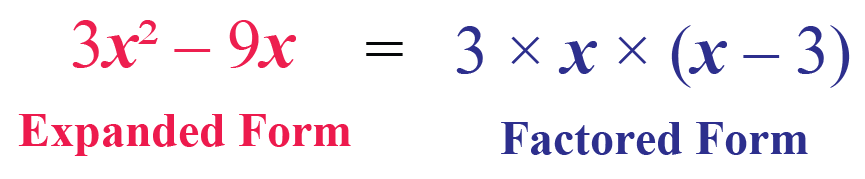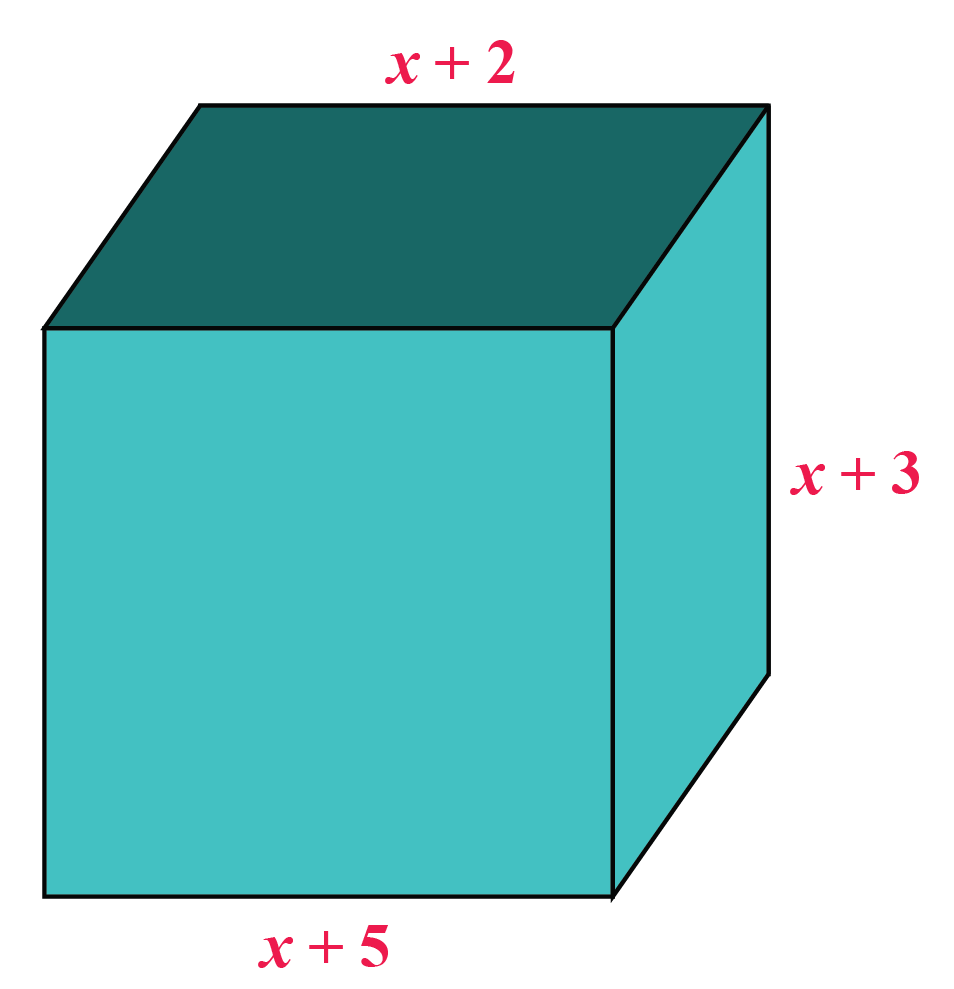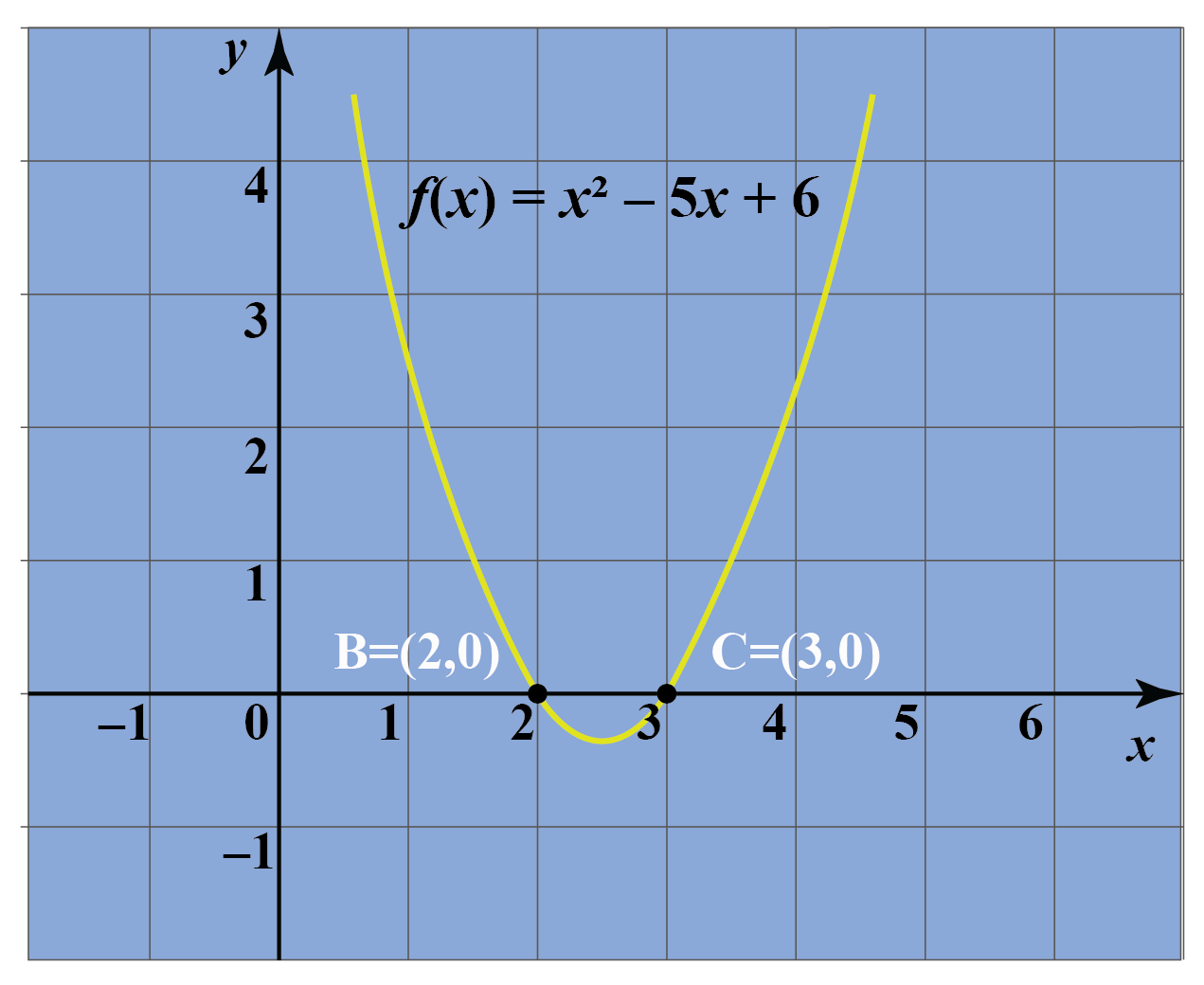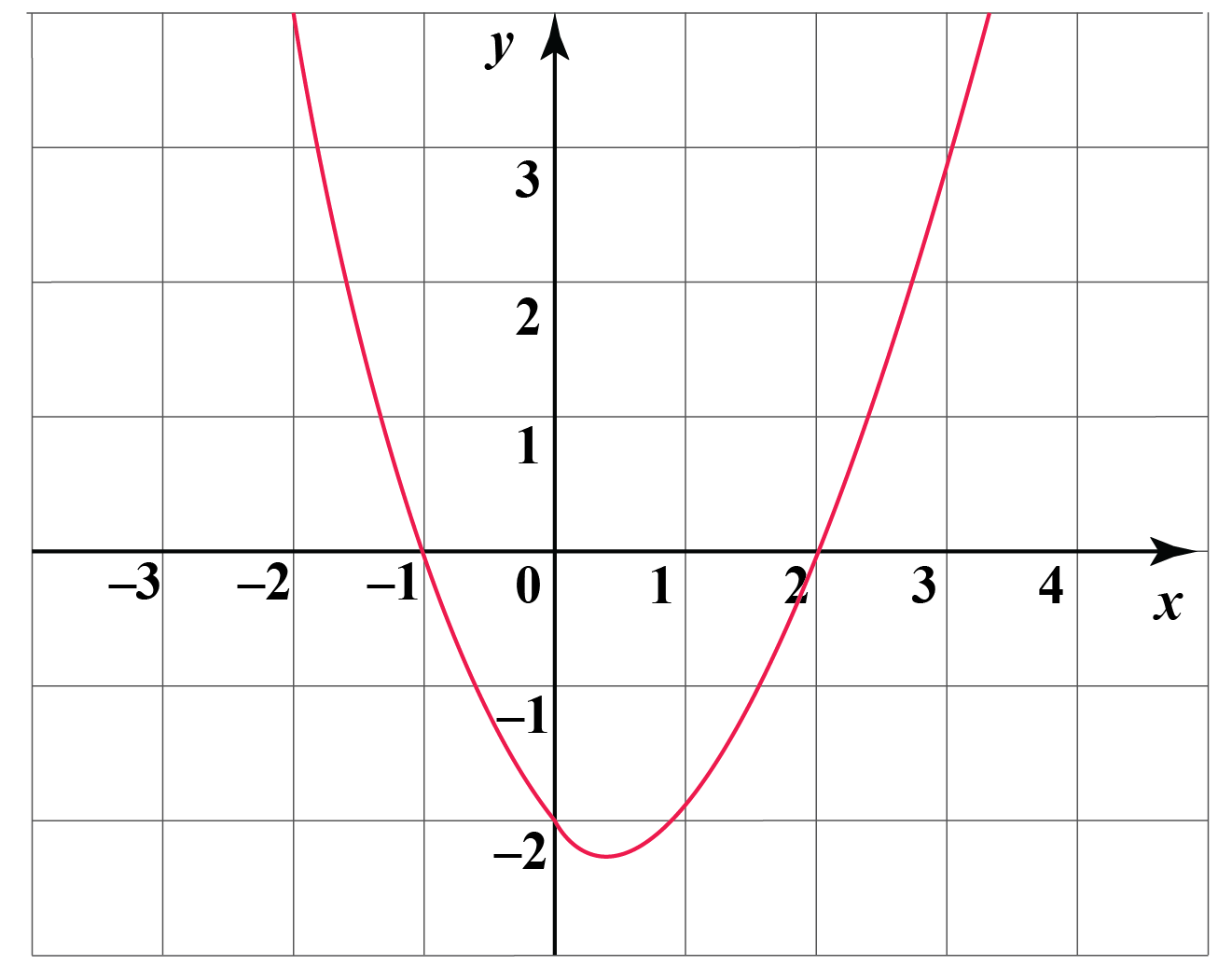# Factored Form

Factored Form

Ryan plans to go on a road trip to Mumbai from Delhi.

He requires to cover a distance of around 1400 km to reach his destination.If his car has an average speed of 70 km per hour, then how many hours will it take to reach Mumbai?

An understanding of factored forms will help us answer this question and help us in working with a factored form calculator.

Let’s learn about factored form, factored form polynomial, factored form parabola, and factored form example and explore factored form calculator.

You can check out the interesting examples to know more about the lesson and try your hand at solving a few interactive questions at the end of the page.

## Lesson Plan

 1 What Is Factored Form? 2 Tips & Tricks on Factored Form 3 Solved Examples on Factored Form 4 Thinking Out of Box! 5 Interactive Questions on Factored Form

## What Is Factored Form?

Mathematically, we can express the above situation as Total distance = Distance covered in 1-hour x Number of hours.

We can calculate the duration by dividing 1280 by 40. The answer will be 32 hours.

$$i.e. 1280 = 40 \times 32$$

What we witness here is the factored form of the number 1280

The process of expressing a given number or algebraic expression as the product of its factors is called factoring.

Consider the factor form of binomial $$3x^2 - 9x = 3x (x-3)$$.Let's get a little deeper into the concept.

Look at the picture below.Hey! Don't start thinking which one out of these is your favorite flavor.

Think about the ingredients which are required to make such yummy ice creams.

Now, we have a polynomial:  $$x^3-4x^2-11x +30$$ which is made up of three binomials:  $$(x-2), (x+3), (x-5)$$.

On multiplying  $$(x-2), (x+3)$$ and $$(x-5)$$, we get the cubic polynomial  $$x^3-4x^2-11x +30$$.

We can also visualize this as a cuboid with dimensions as shown below.\begin{align}\text{Volume of cuboid} &= L\times B\times H\\&=x^3-4x^2-11x +30\end{align}

The factored form of a quadratic equation $$Ax^2 +Bx+C=0$$ can be obtained by various methods.

Depending upon the case, a suitable method is applied to find the factors.

### 1. Finding GCD (Greatest Common Divisor)

When every term of the equation has GCD $$\neq 0$$, then it can be factored by taking out GCD as a common factor.

Example: Observe each term of $$3x^2-6x+12=0$$.

Did you notice that 3 is common to all the terms?

So,

\begin{align}  3x^2-6x+12=0\\\Rightarrow 3(x^2-2x+4)\end{align}

Factors of  $$3x^2-6x+12=0$$ are  $$3$$ and $$(x^2-2x+4)$$

### 2. Using Identity a2 - b2

When the given equation can be expressed in the form a2 - b2, it can be factored as $$(a+b)(a-b)$$.

Example: Consider $$y^2-100$$.

Each of the terms here can be expressed in the form of square.

\begin{align} y^2-100&= y^2-10^2\\&=(y+10)(y-10) \end{align}

Here the factors are $$(y+10$$) and $$(y-10)$$.

### 3. Using Identity (x+a)(x+b)

Sometimes, it is possible to express a quadratic equation in the form $$(x+a)(x+b)$$.

Let's understand this through an example.

Example: $$x^2-5x+6=0$$

There are integers -2 and -3 which on adding give the sum as middle term -5 and on multiplying give the product as last term 6.

So,

\begin{align} x^2-5x+6=0\\x^2-2x-3x+6=0\\x(x-2)-3(x-2)=0\x-2)(x-3) \end{align} Therefore the factored form of \(x^2-5x+6=0 is $$(x-2)(x-3)=0$$.

## Factoring Simulator

Arrange the variables and constants to form a rectangle and find factors of the given quadratic trinomial.

Drag the red dots to move the boxes.

## Benefits of Factoring Quadratic Equations

The factored form of a quadratic equation helps in finding its roots or solutions.

For example: As seen in the previous section, the factored form of $$x^2-5x+6=0$$ is $$(x-2)(x-3)=0$$.

Now, when the product of two terms is 0 it means either of them could be 0.

So,

\begin{align} (x-2)(x-3)&=0\\\Rightarrow (x-2)=0 \,\,&\text{or} \,(x-3)=0 \\x=2 \,\,&\text{or} \,x=3\end{align}

If we plot this on a graph, it will represent a parabola intersecting $$x$$-axis at points 2 and 3Tips and Tricks

While finding the factored form of a polynomial, consider the following points:

1. Check out for any common terms (GCD).
2. Look for any algebraic identities which are applicable.
3. Keep factoring till you reach the simplest form, i.e. the form which is not further divisible.

## Solved Examples

 Example 1

Jimmy has a graph shown below.She wants to find the factored form of the given quadratic polynomial.

How can we help her?

Solution

Jimmy's graph shows a parabola which represents a quadratic polynomial.

As it intersects the $$x$$-axis at points -1 and 2, this means these two numbers are roots of a quadratic polynomial.

Consider the general form $$Ax^2 +Bx+C= (x-a)(x-b)$$, where $$a$$ and $$b$$ are the roots of the polynomial.

Here, $$a =-1$$ and $$b =2$$.

So the factored form will be $$(x-(-1))(x-2)=(x+1)(x-2)$$

 $$\therefore$$, The Factored form is $$(x+1)(x-2)$$.
 Example 2

Sia wants to express $$12 y^2-27$$ in factored form.

What are the steps she needs to follow?

Solution

In $$12 y^2-27$$, the two terms have 3 as their GCD.

\begin{align} 12 y^2-27&= 3(4y^2-9)\\&=3[(2y)^2-3^2]\\&=3(2y+3)(2y-3)\end{align}

 $$\therefore$$ $$12 y^2-27=3(2y+3)(2y-3)$$
 Example 3

Find the roots of $$x^2-9x+20$$.

Solution

To find the roots, consider$$\, x^2-9x+20=0$$

Let's find its factored form.

Middle term = -9 and last term = 20

So the numbers which give sum= -9 and product= 20 are -4 and -5

\begin{align} x^2-9x+20&=0 \\ x^2-5x-4x+20&=0\\x(x-5)-4(x-5)&=0\x-5)(x-4)&=0\\\therefore x=5 \,or\,x&=4\end{align}  \(\therefore 4 and 5 are the roots of $$x^2-9x+20$$
 Example 4

Express $$\dfrac{4m^2}{9}-100$$ in a factored form for Leena.

She wants to find the answer before going to school the next day.

Solution

The two terms have GCD as 4

\begin{align} 4 \times(\dfrac{m^2}{9}-25)&=4 \times[(\dfrac{m}{3})^2-5^2]\\&=4 \times (\dfrac{m}{3}+5)(\dfrac{m}{3}-5)\end{align}

$$\therefore$$ The factored form is

 $$\therefore$$  $$4\times (\dfrac{m}{3}+5)(\dfrac{m}{3}-5)$$Think Tank
1. What will be the graph of the equation $$x^2-4$$? What will be its $$x$$-intercept?
2. Which method will be suitable to find factored form of $$\sqrt3x^2+10x-8\sqrt 3=0$$?

## Interactive Questions

Here are a few activities for you to practice. Select/Type your answer and click the "Check Answer" button to see the result.

## Let's Summarize

We hope you enjoyed learning about Factored Form with the simulations and practice questions. Now, you will be able to easily solve problems on factored form polynomial, factored form calculator, factored form parabola, and factored form example.

At Cuemath, our team of math experts is dedicated to making learning fun for our favorite readers, the students!

Through an interactive and engaging learning-teaching-learning approach, the teachers explore all angles of a topic.

Be it worksheets, online classes, doubt sessions, or any other form of relation, it’s the logical thinking and smart learning approach that we, at Cuemath, believe in.

## 1. What is a fully factored form?

A fully factored form means the given number or polynomial is expressed as a product of the simplest possible form.

For example, if we write $$12 y^2-27= 3(4y^2-9)$$, then it is not considered as fully factored form as $$(4y^2-9)$$ can be factored further.

So, $$12 y^2-27=3(2y+3)(2y-3)$$ is its fully factored form.

## 2. How do you write a factored form?

To write a polynomial in factored form, it must be expressed as a product of terms in its simplest form.

The terms could be constant or linear or any polynomial form which is not further divisible.

## 3. How do you find the factored form of a quadratic equation?

The factored form of a quadratic equation can be obtained by various methods.

The most common method is finding GCD and using a suitable identity.

More Important Topics
More Important Topics